Perceptions of Physical Reality - 3 by Raj Vatsya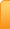Channels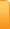Analysis
Cartoons
Education
Environment
Opinion
Photo Essays

Random Thoughts

Architecture
Astrology
Ayurveda
Buddhism
Cinema
Culture
Festivals
Hinduism
History
People
Places
Sikhism
Spirituality

Health
Parenting
Perspective
Recipes
Society
Teens
Women

## Creative Writings

Book Reviews
Computing
Humor
Individuality
Literary Shelf
Memoirs
Quotes
Stories
Travelogues
Workshop

Perceptions of Physical Reality - 3
by Dr. Raj Vatsya

Classical Theories - Forces

Present article is the 3rd one in a series, which continues from the 2nd: Perceptions of Physical Reality/ Classical Theories – Motion of Particles, cited here as [II]; which together with the article cited in it should be checked for explanations of some of the concepts appearing here. In [II], I had discussed the motion of particles in the framework of pre-quantum modern classical theories, where the concept of force was introduced, which determines the trajectory of a particle and other related quantities. The issues pertaining to the force are discussed in the present article.

Classically, the force was assumed to arise out of a body’s interaction with the other bodies. If a particle is in a non-inertial frame of reference, it experiences an alteration in its state of motion, which does not arise out of its interaction with any other body. This type of alteration was also attributed to a “virtual” force. Thus, every motion deviating from a rectilinear one with constant velocity was attributed to some force. In the classical physics, initially, three types of the forces arising out of a body’s interaction with the other bodies were known: Gravitational; Electric; and Magnetic. The electric and magnetic forces were later formulated as the components of a single force: Electromagnetic. This leaves only two fundamental forces. In this article, we discuss the issues pertaining to these forces. Except for the virtual forces, all other forces arise out of these fundamental forces, e.g., the frictional and mechanical forces are described in terms of the electromagnetic forces. A separate discussion of the virtual forces would not add much to our understanding at this stage. The present description may deviate from the others. Purpose here is to explain the underlying concepts rather that to follow a standard course.

GravitationIf a pebble is released in air above ground, it falls to the ground. As it is released, it is at rest. According to the first law of motion, it would maintain its state of rest, remain suspended in air, unless there was a force impressed upon it. Since it falls to the ground vertically pointing to the center of Earth where Earth’s mass can be assumed to be concentrated, it is accelerated towards the center. From Newton’s second law of motion, the direction of the force applied and the acceleration is the same. Thus, the center of Earth must be the source of some force forcing it to accelerate towards the center. This force is called the gravitational force. According to Newton’s third law, the pebble applies equal and opposite force on the Earth. Thus, the Earth also “falls” towards the pebble. However, due to the mass of pebble being miniscule compared to that of the Earth, an alteration in its state of motion is not noticed.

The concept of gravitation developed out of the studies of the planetary motion. Kepler’s first law of planetary motion states: The orbit of every planet is an ellipse with the Sun at one of its two foci. Before Kepler, it was believed that the planetary orbits were circular. An ellipse is roughly speaking a circle stretched along its diameter making its center into two foci and instead of a constant diameter, two axes, major and minor, perpendicular to each other. A circle is just a special type of ellipse. Details pertaining to the ellipses and planetary motion can be found at a number of websites.

My purpose here is to explain the basic concepts involved in understanding the gravitation that are not readily available elsewhere.

To understand the behavior of the orbiting planets, consider the pebble now propelled horizontally with a non-zero velocity. With a low velocity, the pebble travels along a parabolic trajectory to land on the ground. As the velocity is increased, the pebble lands farther and farther from where it does at the lower velocities. As the initial velocity of the pebble is increased, increasing this distance the trajectory bends noticeably towards the Earth’s center since the pebble experiences the gravitational force towards the center of Earth at every point of its trajectory. As the velocity is increased further, there will reach a point when the pebble would have covered the Earth, all ground ahead of it; it would not touch the ground in one go and thus, would spiral to the ground. When a critical velocity is reached, the pebble would maintain its height going into an orbit around the Earth, perpetually falling to it but never reaching its surface. Going around in a circle is a perpetual fall to its center. Allow me to insert one of my short poems here:

Cycle

Step in life at birth
was soon consumed by perpetual fall
Center is still far as ever

Going into future I meet myself as stranger
as shadow of past
over and over

I must be riding around in circles.

Returning to the motion, we considered the motion of a pebble propelled horizontally. In general, the shape and size of the orbit would depend on the distance between the Earth and the orbiting body, pebble, and its initial velocity, which includes the direction with respect to the horizontal. In case of the planets, it is an ellipse in general with the center of gravity being at the Sun on one of its foci. The planets were propelled at the time of their creation believed to be at the time of the big bang. Since the velocity is defined by its magnitude, i.e., its speed, and its direction, a body, even if it is moving with a constant speed but along a non-rectilinear trajectory experiences acceleration. The magnitude and direction of this acceleration can be calculated from the geometrical considerations alone. In case of the planets, the acceleration is in the direction of the Sun.

Based on such considerations, Newton suggested that gravitation is universal in that all bodies apply an attractive gravitational force upon each other. By studying the motion of planets, particularly by comparing the acceleration of the moon in Earth’s orbit with that of a body like a pebble on Earth, Newton developed the inverse square law: The gravitational force between two bodies is inversely proportional to the square of the distance between them. For example, if the distance between two bodies is doubled, the force between them reduces to a quarter.

Although credited to Newton, some other authors are said to have arrived at the inverse square law before or independently. In particular Robert Hooke accused Newton of plagiarism by claiming that Newton had plagiarized the essential concept from him. What did Newton owe to Hooke, if any, is still a subject of discussions exciting some controversy. On the other hand, Newton did accept and acknowledge that Hooke, Wren and Halley had separately appreciated the inverse square law in the solar system.

As indicated above, Newton had arrived at the inverse square law by comparing the accelerations of two bodies with different masses in the gravitational field of the Earth. Aristotle had theorized that the bodies of different masses released at the same point would fall to the ground at different times, the heavier one falling faster proportionate to its mass. This view persisted until Galileo’s famous experiment at the leaning tower of Pisa. Galileo released two bodies with significantly different masses from the top of the tower. It is said that both bodies hit the ground at the same time thereby proving that their accelerations and velocities at each point of their respective trajectories were equal. This experiment is repeated regularly commemorating the original one and the bodies fall to the ground at different times although nowhere near being what the earlier views would suggest. This is likely what happened in Galileo’s original experiment also. Galileo did discuss this difference and explained it by considering the friction between the air and the bodies. Recent experiments on the surface of the Moon, where there is no air like substance, with a hammer and a feather confirm it with about complete accuracy. Newton of course was aware of this result.

According to the second law of motion, the mass of a body multiplied by its acceleration is equal to the force impressed upon it. Thus, a more massive body would experience proportionately lower acceleration in the same force field, e.g., if the mass is doubled with the force being the same, the acceleration would reduce to its half. Thus, the mass acts as the inertial constant, i.e., resisting the motion. For this reason, the mass was also call the constant of inertia. As Galileo’s experiment shows, two bodies of different masses experience the same acceleration in the Earth’s gravitational field. This can happen only if the force increases proportionately as the inertial mass is increased. For example, as the mass is doubled, the force must also double to keep the acceleration unchanged. Consequently, the gravitational force must be proportional to the mass of the accelerated body.

Thus, the mass of a single body plays two completely opposite roles. As an inertial constant, it impedes the motion giving it the name “inertial mass.” As a part of the gravitational force, it assists the motion. In this role, the mass is called the “gravitational mass.” Numerically, both of the masses are equal but conceptually, they are completely opposite. Consider again a pebble falling to the Earth. In our illustration, the gravitation force experienced by the pebble is directly proportional to its gravitational mass. However, the Earth also falls into the pebble. Applying the same argument to the Earth, the gravitational force between the pebble and the Earth should also be proportional to the mass of Earth. Carrying this argument to universality, each body in a pair of two applies a gravitational force on the other, which is directly proportional to their gravitational masses and inversely proportional to the square of the distance between them. The proportionality constant called the universal gravitational constant was measured by Cavendish later.

In the example of the pebble and Earth, we have concentrated on the motion of pebble. In this consideration, the gravitational mass of the pebble is called the “active gravitational mass,” and the mass of Earth is called the “passive gravitational mass.” Conceptually, there is no basic difference between the two gravitational masses. It is just that if the study is focused on a particular body, its gravitational mass is the active one, and the other one is passive. If in the study, the focus is on the motion of the other body, its gravitational mass would be the active one and the first one’s would be passive.

ElectricityEffects of electricity were noticed well before its understanding developed in modern times, e.g., in the form of shocks from the electric fish, which the ancient Egyptians called the “Thunderer of the Nile.” Ancient cultures in the Middle East had discovered that if a rod of amber was rubbed with cat’s fur, it attracted light objects like hair. Thales of Miletus studied this effect in more detail around 600 BC and theorized that the rubbing rendered amber “magnetic” enabling it to attract light objects. Knowledge of the magnetic material that needed no rubbing existed already. Thales’ conclusion was later found to be false as the rubbing deposited an electric charge on amber and an opposite charge on fur. In fact rubbing transferred some charge of one kind from one object to the other rendering them both oppositely charged. Labels “positive” and “negative” were assigned to opposite charges arbitrarily. It did not matter which charge was called positive and which one negative as far as the understanding of nature goes. In any case, the like charges repel each other and the opposite charges, attract, with the force being inversely proportional to the square of distance between the charges and directly proportional to the charges. In comparison, the gravitational force is always attractive. A better understanding of electricity was not developed until William Gilbert’s studies who also coined the word “electricus,” meaning “like amber;” around 1600. Benjamin Franklin conducted extensive studies on electricity around 1750. He is said to have attached a metal key to a dampened kite string and flown the kite in a storm threatened sky to produce sparks jumping from the key to the back of his hand to demonstrate that lightening was electric in nature.

Major advance was made with the Alessandro Volta’s construction of the voltaic pile with alternating layers of zinc and copper, which later developed into the Voltaic cell. The pile was used to produce the electric current, which is a stream of flowing charges, i.e., the moving charge. In this pile, as well as in the Voltaic cell, the positive charge flows from copper to zinc. This is taken to be the direction of current. In fact, it is the negatively charged electrons that flow from zinc to copper. Thus, the reverse of the original convention of naming the charges would have been more in line with this phenomenon. However, as mentioned before, this would not change our understanding of nature. Close contact between zinc and copper often achieved by a mediating agent enables the electrons to transfer from zinc to copper due to intermolecular processes.

In the early nineteenth century, Machael Faraday introduced the concept of electric field. An electric field is created by a charged body in the space surrounding it that results in a force exerted on any other charges placed within the field. Thus, it is the field in the vicinity of a charged particle that exerts the force on the particle. This mitigates, somewhat, the tenuous concept of the action at a distance but not at a fundamental level.

MagnetismAs mentioned above, Thales of Miletus, around 600 BC, considered an electric effect a magnetic one as the knowledge of the magnetic material existed at the time, which he discussed in his scientific discourses. Around the same time, an Indian surgeon, Sushruta used magnet for surgical purposes. Later around 400 BC Chinese naturalists noticed that the lodestone attracts iron, which is a magnetic effect. Around 1050 AD a Chinese scientist, Shen Kuo, wrote about the magnetic needle compass, which improved the accuracy of navigation as its one pole points to the North and the other, to South. A magnet has two poles on its opposite ends, one pointing to the North and the other to the South. William Gilbert in about 1600 concluded from his experiments that the Earth itself is a giant magnet with the magnetic poles about the geographical North and South poles but do not coincide with them, which he used to describe the behavior of the needle of compass. Opposite poles attract each other and the like, repel, aligning the magnetic compass in North-South position. The force between the poles is also inversely proportional to the square of distance between the poles and directly proportional to the pole strengths. As in the case of electric charges, Faraday’s concept of the field applies to the magnetic forces also. However, while the electric charges can exist as single units, the magnetic poles come in pairs and the field-lines, or the lines of force originate at one of the poles, conventionally taken to be the North Pole and terminate at the South.

The areas of electricity and magnetism are quite wide in themselves. Purpose here is not to cover the topics of physics in any detail; it is to impart an understanding of how our perceptions of physical reality developed and what they are at a basic conceptual level. From this point of view, not much more is to be gained by describing these topics in further detail. Electric forces and magnetic forces were later understood to be the components of a single force, electromagnetic, which is described next.

Electromagnetism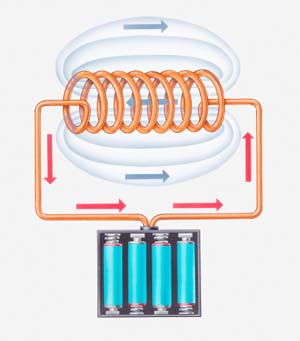Studies of Hans Christian Orsted and Andre Marie Ampere around 1820 resulted in the recognition that the electric and magnetic fields are intertwined. It was noticed that a compass needle was affected, moved, by an electric current flowing in a wire close to the compass. This could happen only if the electric current produced a magnetic field about the wire. Ampere then formulated his law relating precisely the current and the field associated with it. James Clerk Maxwell, in about 1860, adjusted this law by introducing the concept of displacement current, which is the rate of change of the electric field. The concept of the displacement current was in fact a quite clever and radical concept. Maxwell had introduced it on mathematical grounds, which although can be understood in physical terms. There are other instances in physics when just a mathematical consideration has resulted in a breakthrough and predicted physical phenomena, which were later verified. Mathematics is a concoction of human mind; by itself it has nothing to do with reality, while describing reality is the basic purpose of physics. This suggests that mind does impact upon the reality rendering it somewhat subjective as a number of natural philosophers have argued throughout the ages (I).

If a copper wire is coiled around an iron rod with an electric current passing through it, the rod becomes magnetic capable of attracting other iron pieces. This can be described in terms of Ampere’s law. Around 1831 Faraday formulated a parallel law on the basis that a varying magnetic field through a closed loop of wire produces an electric current in the wire. It is said that Faraday argued that if an electric current through a coil results in a magnet along its axis, then a magnet through the coil should produce an electric current in the coil. He experimented with this view for about four years. The expected phenomenon never occurred. One day, frustrated Faraday threw the magnet through the coil in anger. The needle of the current measuring instrument connected to the wire moved. Then he realized that it was not the static magnetic field that produced the current, even though a constant current produces a static magnet; it is the varying magnetic field that produces the current. Further experiments lead to the formulation of his law.

The above laws, Ampere’s and Faraday’s, establish clearly that the electric and magnetic fields are intertwined in the form of the electromagnetic fields. Gauss’s law relating the electric charge with its field with a similar law for the magnetic field already existed. All four laws, Ampere’s, Faraday’s and both Gauss’s laws, constitute Maxwell’s equation formulating the classical electromagnetism. We see gadgets all around us based on the electromagnetic phenomena, which have transformed our lives, e.g., the electric motors and generators.

A charge moving at a constant velocity is a constant current, which according to Ampere’s law produces a magnetic field. Thus, an accelerated charge produces a varying magnetic field. From Faraday’s law, varying magnetic field produces an electric field, which impacts upon the magnetic field. Thus, an accelerated charge produces interlinked varying electric and magnetic fields. While it requires a careful analysis of Maxwell’s equations, this phenomenon provides a basic understanding of the electromagnetic waves. A field can get dissociated from its source, the charge, and travel independently in vacuum. In an electromagnetic wave, the electric field oscillates in one plane and the magnetic field in a plane perpendicular to the electric; the electromagnetic energy travels in the form of a wave in a plane perpendicular to both planes. This classifies them as the transverse waves like the waves on the water surface where the water particles oscillate in the vertical direction and the energy travels with the wave perpendicular to it, in the horizontal. In contrast, the longitudinal waves, e.g., the sound waves, travel in the direction of the oscillations of the particles producing them. This is another example where a physical phenomenon, electromagnetic waves, was predicted by mathematical analysis, which was later verified experimentally. Heinrich Hertz in the late 1980s produced the electromagnetic waves in laboratory and determined their speed, which was found to be the same as that of light in vacuum. On this basis it was theorized and later confirmed that light is a form of the electromagnetic waves.

Light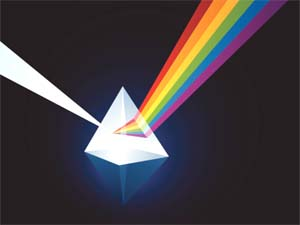Although the theories of light existed in ancient times, its understanding in line with the modern thinking is more recent. In about 300 BC, Euclid studied the properties of light more systematically. He postulated that light travelled in straight lines and studied them mathematically. Couple of millennia later, in 1662, Fermat formulated his principle of least time, which assigns straight lines to a ray of light in free space (II). There were flaws in Euclid’s theory as a whole and therefore it was not accepted. Descartes considered light a mechanical property of the luminous body. In the 1630s, he developed a theory of refraction of light assuming, incorrectly, that light travelled faster in a denser medium than in a less dense medium. In spite of its flaws, it had sufficient correct thinking in it to be regarded as the start of the modern physical optics.

Whether the light is a stream of particles or a waveform has been a topic of inquiry and controversy from the early times. Newton assumed that light was composed of tiny corpuscles emitted from a source. One of Newton's arguments against the wave nature of light, the view forwarded by some scientists, was that the waves were known to bend around obstacles, while light travelled only in straight lines. He did, assume however, that a light particle could create a localised wave to explain the observed phenomenon of diffraction of light. As Descartes, he assumed that light travelled faster in a denser medium.

If two beams of particles originating at two sources overlaps on an observation screen, then the total particle density would be equal the sum of the two densities. However, if two identical waves overlap with each other, the observation is different. If the crest of one wave falls on the crest of the other, and trough on trough, i.e., the waves are in the same phase, they interfere constructively, and the amplitude doubles. If the crest of one falls on the trough of the other, i.e., they are out of phase by 180 degrees, they interfere destructively, and the two waves cancel each other out with the resulting amplitude being zero. The combined amplitude varies in between, depending on the phase difference. Thus the resulting amplitude can be anywhere between zero and double. This is one significant feature distinguishing the particles and waves.

If a lens is placed on a glass plate, then an incident beam of light is reflected by the upper surface of the lens and from the plate. The reflected beams overlap with each other. Robert Hooke conducted this experiment and observed the rings of light, i.e., alternating bright and dark circles around the central spot. This can only happen if the light is a waveform. The bright rings form where the beams interfere constructively and the dark, where they interfere destructively. The phase difference depends on the difference in the distance travelled by each wave. Newton could not explain this phenomenon on the basis of his corpuscular theory. However, his reputation and power he had accumulated in himself, which he used to push, impose, his views, helped his corpuscular theory to hold during the 18th century and the rings are still called Newton’s rings.

Although the works of Robert Hooke, Christian Huygens and others contributed to describing the light as a waveform, it was the double slit experiment of Thomas Young in 1800, which finally did establish the wave theory of light. In a double slit experiment, a coherent beam of light emitted by one source is divided into two identical wavelets by two slits, A and B, in a screen and observed on an observation screen, as shown in the figure below.

The two identical wavelets interfere with each other showing the alternating bright and dark spots. The phase difference depends on the distance between the slits and the spot on the screen. The bright spots result from the constructive interference and the dark, from the destructive.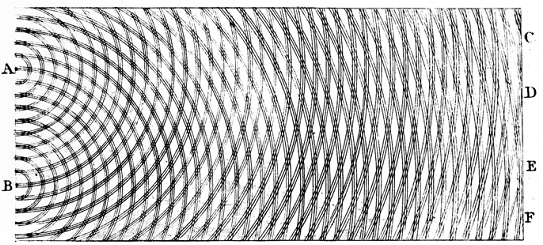Image courtesy Wikipedia.org

Young’s theory was not well greeted in England for it contradicted Newton’s. However, there was no such inhibition elsewhere. A Frenchman, Fresnel, conducted further studies and aided by Poisson’s mathematical analysis helped the wave theory overturn the corpuscular theory. As indicated above, comprehensive work on electromagnetism resulting in Maxwell’s equations together with the electromagnetic waves, which were produced by Hertz, placed the wave theory of light on a strong footing. It should be mentioned that while Newton's corpuscular theory required that light travel faster in a denser medium, the wave theory implies the opposite, which is now known to be true.

A wave needs a medium to travel, e.g., the waves on the surface of water and the sound waves. However, there is plenty of vacuum in between the Earth and the Sun, for example, and the light travels through it. Thus, light can travel in vacuum, where a wave cannot. To correct for this weakness of the wave theory of light, existence of a hypothetical substance “luminiferous aether” was proposed by Huygens in 1678. What is aether? What the vacuum is made of. This is clearly a tenuous assumption. Assumption of aether also violates the Galilean principle of relativity as the frame attached to it would be at absolute rest while the Galilean relativity principle considers all inertial frames equivalent.

Concluding Remarks

In the classical physics, which prevailed from the time of Galileo to the end of the nineteenth century, the concept of force was developed, postulated, to describe the motion of material bodies deviating from a constant velocity. The gravitational force that exists between every pair of two massive bodies is always attractive. The electric force is exerted by one charged body on the other charged body; like charges repel each other and unlike ones attract. Similarly, the like magnetic poles repel each other and the opposite ones attract. The magnetic poles always exist in pairs of opposite poles. Electric current is just a stream of the moving charges. Electricity and magnetism were later combined into electromagnetism, which acquired a significance of its own. Light was understood in time to be the electromagnetic waves, which have infinitely many wavelengths. Visible range covers a narrow wavelength range.

Action at a distance was assumed to exist to reconcile with the fact that two bodies separated in space can exert force on each other, which is a tenuous assumption. In electromagnetism, this assumption was mitigated somewhat by introducing the concept of the field. The field disturbances could travel as the electromagnetic waves. To explain the fact that light can travel in vacuum, existence of aether was assumed, which is as unsettling an assumption as the action at a distance. As the theories of physics are improved upon for one reason or the other, they often replace one tenuous assumption by the other and at times introduce their own. In spite of this, they succeed in describing the nature but always to a limited extent.

A basic tenet of the classical theories is that they are deterministic. In case of the motion of a particle, its trajectory is exactly determined and all physical observables, e.g., its momentum and energy are also precisely determined. In case of the waves, their propagation and the associated physical observables are also precisely determined. Such is not the case with the quantum theories developed later, which is the currently prevailing theory.

Images (c) Gettyimages.com

05-Oct-2013
More by :  Dr. Raj Vatsya

Top | Education

Views: 3501      Comments: 5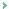To P. Rao - It is true that not everything was 'created at the time of Big Bang.' The processes were set within a microsecond after the Big Bang. Events are taking place as a result of those processes and their outcomes. Then defining the time of Big Bang is also difficult for the question remains; What was the duration of Big Bang; when could it be considered completed? Also, the Big Bang Theory itself is still controversial. In writing such an article, one relies on the widely accepted wisdom.

Raj Vatsya
10/04/2018 12:16 PMTo P. Rao - Sorry not to have noticed your comment sooner. Elliptic motion is also perpetual fall, and free fall at that; the orbiting body falls to one of the focal points, the one which is the center of force. This covers the circular motion also as a circle is an ellipse, with both focal points at the center. The poem quoted is not meant to describe physics; it pertains to a human situation, feeling. I quoted it for its similarity with a physical situation.

Raj Vatsya
10/04/2018 09:29 AMI learned here that "Going around in a circle is a perpetual fall to its center." This is not apparent to a non-physicist. Thanks for explaining what is circular motion.About the statement, "In case of the planets, it is an ellipse in general with the center of gravity being at the Sun on one of its foci. The planets were propelled at the time of their creation believed to be at the time of the big bang." I thought the solar system along with the planets was created much later than the big bang.

P. Rao
07/25/2015 11:23 AMAuthor’s correction.Definitions of the active and passive gravitational masses given in the last paragraph of the first section, “Gravitation,” are opposite of the conventional ones. Since this paragraph is of no consequence here, it can be ignored. Alternatively, this paragraph can be replaced by the following:“In the example of the pebble and Earth, we have concentrated on the motion of pebble, i.e., the pebble responds to the gravitational field created by the Earth. In this consideration, the gravitational mass of the Earth is called the “active gravitational mass,” and the mass of the pebble is called the “passive gravitational mass.” If the response of the Earth is studied in the gravitational field created by the pebble, then the gravitational mass of the pebble is active, and the gravitational mass of the Earth is passive. Thus, these definitions are basically for convenience of description, not of any conceptual consequence.”If and when there is a danger of confusion, attempt will be made to provide sufficient explanation to clarify the concepts.

Raj Vatsya
10/26/2013 11:02 AMJust a comment that the curves of motion described as circle, ellipse and parabola,and hyperbola, are conic sections, that is, planes cut through a three-dimensional cone. The fact that paths traced by objects responding to the force of gravity assume the shape of ellipse, as in planetary motion, and parabola as in projectiles, that is, of the relevant conic section, is too close to be mere coincidence. .One observes that in the motion of planets, for example, the path coincides with the conic section of an ellipse, with a real and virtual focus, the real focus occupied by the body being orbited by the planet. In strict accordance to theory, equal areas are swept out by the planet in respect of each focus. But the planet's acceleration in proximity with the real focus is what causes it to 'escape' into a wider arc. Once it approaches the virtual focus, which doesn't physically exist, in a state of decceleration, it yet sweeps round it on the return to the real focus locked into the precise symmetry of the ellipse!

rdashby
10/08/2013 20:46 PM

Name *
Email ID
(will not be published)
Comment *
Characters
Verification Code*
Please fill the above code for verification.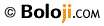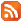RSS Feed Home | Privacy Policy | Disclaimer | Site Map